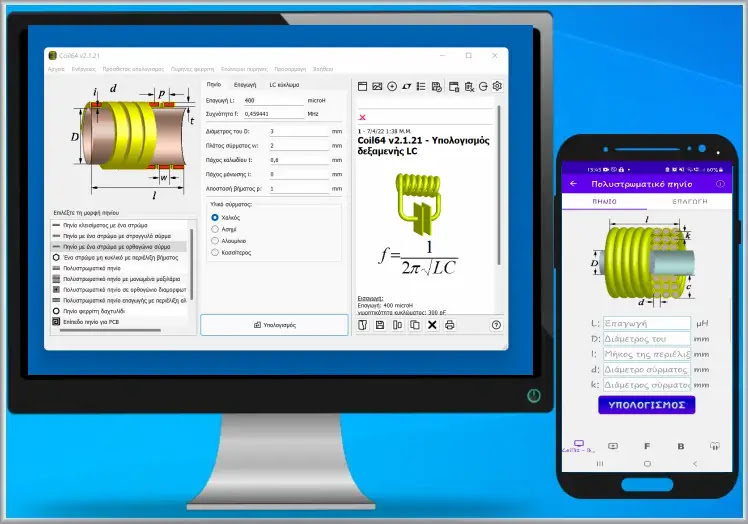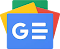## Saturday 4 March 2023Coil32 is probably the best free app out there for electronics lovers. Which in particular is a coil calculator that allows calculation on single layer and multi layer air core inductors, air core spiral wound inductance, ferrite ring inductors, planar coils on PCB and many other variations.

The software allows the calculation of coils in all variations, and it is possible to calculate its inductance if the number of turns is known or to calculate the number of turns of the coil if its inductance is known.

The program calculates the induction of different types of coil design. It is possible to choose an optimal variant, use the available coil modulator or recalculate the inductor in other dimensions. Coil64 allows the estimation of the Q factor, the self-tuning frequency and the self-capacitance of the RF single core air core inductor.

Coil64 allows estimation of the Q-factor and self-capacitance of a toroidal ferrite inductor at RF. It is possible to calculate the length of wire needed to wind a single layer, multi-layer coil and the coil on a ferrite ring.

The results of all calculations are summarized in a common text box, from where they can be saved to a file or printed. For planar coil inductors on the PCB, the program asks for the optimum sizes for the highest Q-factor. It is possible to use metric or inches as units of measurement. Support AWG gauge or metric wire.

OPERATING SYSTEM: Windows, AndroidFollow us on Google News